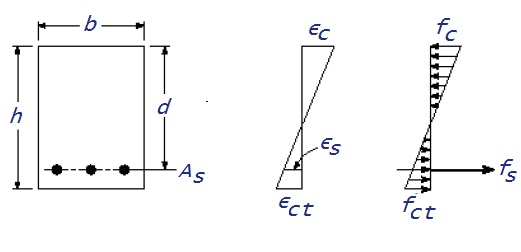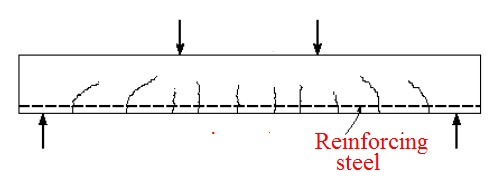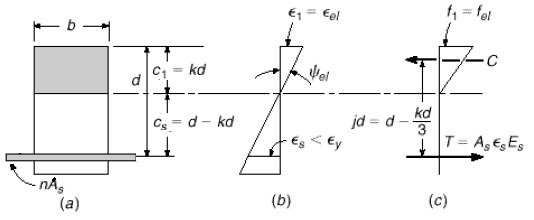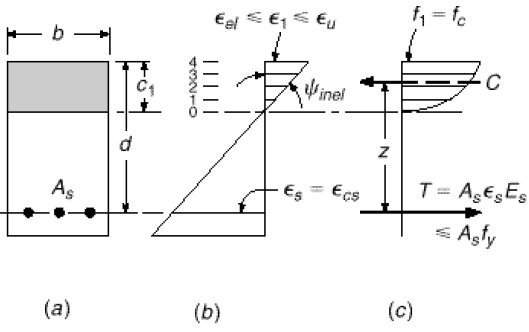Foundation, Concrete and Earthquake Engineering

## Curvature

Curvature is defined as change of angle per unit length along the axis of a object under the action of bending loads at any given section.

## Moment

Moment (force) is a magnitude of tendency to cause an object to rotate with respect to a specific axis or point under the action of a force. Force is included here as it is related to the derivation of this relationship; moment may be of other physical quantity like charge, mass etc.To produce any significant value of moment, the force which result rotation, must be placed in such a way that this will initiate twist in the body. This phenomenon only happened when line of action of force is not colinear with the centroid of respective body.

The magnitude of moment of force about an axis or point has direct relation to the distance between line of action of force and axis about which it rotates. This distance is called moment arm. Moment arm (often called lever arm) is measured orthogonally between line of action of force and center of moment. If force is applied obliquely, only perpendicular component will produce moment.

Moment= Force X moment arm

M= F X d

## Bending moment

When external force (s) or moment (s) is/are applied on an element resulting it to bend, as a reaction bending moment is induced. Bending moment at any section of a structural element can be defined as the summation of moments about this section produced by all external forces that act on one side (either right or left) of this section.

The internal reactions of cross-section of a structural element can be taken as combination of resultant force and resultant couple. To have equilibrium, moment produced by external loading (force and moment) must be counteracted by couple provided by internal loads. The result of internal forces is termed as shear force or normal force (depending on plane of action) and resultant of internal couple is termed as bending moment.

M=∑ML =∑MRFigure-1: Curvatures correspond to bending moments
ML stand for bending moment calculated based on loads that act on left side of the section and MR stand for that at right side of section.

Positive bending moments will produce bending of a beam to concave upward i.e. beam curves downward at the middle (known as sagging); Whereas negative bending moment will result hogging of a beam i.e beam curves upward at the middle.

### Modulus of rupture

Modulus of rupture is very common term in concrete engineering. It is also known as flexural strength or transverse rupture strength (fr). It is the stress just before yielding of a material in flexure test. It represents the maximum stress that a material can experience at the moment of yielding; so the unit of stress is the measuring unit of modulus of rupture.

A homogeneous object (i.e. object consist of single materials) like steel rod or wooden beam will subject to variable stresses throughout its depth under normal service loading. At the edge of concave face (inside of bend) of an object, the stress will reach maximum value and its nature will be compressive. At the edge of convex face (outside of bend), the stress will reach maximum value and is of tensile stress. The inner most and outermost edge of a beam where maximum stresses are induced are termed as extreme fiber. Most of the materials like concrete, it is observed to suffer tensile failure; so the maximum value of stress that can withstand before its failure is known as flexural strength or modulus of rupture of that object.

Flexural stress can be determined by σ = My/I            ..(1)

When stress of extreme fiber need to be determined y is replaced by c where C is distance between neutral axis and remotest fiber.

σ = MC/I            ..(2

Equation (1) and (2) can be used to determine flexure stress of a beam under rupture in testing machine. As at that stage proportional limit of material is exceeded, the stress found in this equation is not the actual stress; but the imaginary stress obtained with this method is known as modulus of rupture. This property of material is used to make comparison of ultimate strength of flexure members of various materials and sizes.

### Bending stress and shear stress:

Internal forces acting on any cross section can be resolved into two components; tangential and normal to that section. Of these components, that act normal to section are called bending stresses which will produce compression on one side and tension on other side. The function of bending stress is to counteract bending moment.

The components that are tangential to the section are called shear stresses, the functions of which are to resist shear or transverse forces.

### Transformed concrete section

When stress in concrete section is low (i.e. ≤f’c/2), concrete is found to act more or less elastically; this means stress is nearly proportional to strains Figure-2 shows the line d which represents this behavior with small error under both slow and fast loading. At this stress, normal weight concrete shows strain of the order of about 0.0005, whereas steel behaves elastically nearly at its yield points (60 ksi) which may be represented by a strain 0.002 (much greater than concrete).Figure-2: Stress-strain curve of concrete and steel

Form the assumption of prefect bond, as compression strain in concrete is equal to compression strain in steel at a given load,

Where n=Es/Ec =Modular ratioFigure-3:Transformed section of reinforced concrete section under axial compression
With reference to Figure-3 area of transformed section can be determined as

At=Ac + nAst

=Ag-
Ast+nAst

=
Ag+Ast(n-1)

Where
Ag=gross area of concrete section
Ac= net area of concrete
Ast= sum of areas of all reinforcing bars

This means an addition of Ast(n-1) is required.

Before development of tensile crack (stress ≤f
r) distribution of stress and strain is almost equivalent to elastic and homogeneous beam; except presence of steel reinforcement. From equation (3) it can be concluded that within elastic range, steel is subjected to n times stress as that of concrete. This fact can be used to determine fictitious section only consists of concrete by replacing steel-concrete cross-section which is called transformed section. In this section, actual area of steel is replaced by equivalent area of concrete, nAs.

Reinforced concrete members are non-homogeneous as they consist of two completely different materials; so the procedures to analyze reinforced concrete members are not the same as that of methods used in analyzing or designing of members of homogeneous materials like wood, steel or other materials. The basic principles considered, though, essentially identical. This additional area of concrete is added at the level of reinforcing steel as shown in figure-3.

### Application of transformed section

Once transformed concrete section is obtained, the following calculation becomes more convenient

• Analysis of homogeneous elastic beam can be applied to concrete beams

• Section properties can be calculated easily as per usual manner (i.e. position of NA, moment of inertia; section modulus etc.) which will furnish particular stresses like bending stress and shear stresses.

### Significance of moment-curvature relationship:

Although this relationship is not included in ACI code and also is not required evidently in general design, the curvature resulted from moment put on a particular section of beam under full extent of loading leading to failure is required in different contexts. This relationship is the base for studying

• Ductility of member
• Understanding the formation of plastic hinge
• Calculating redistribution of elastic moments which may occur in reinforced concrete members before collapse.

### Development of moment-curvature Plot:Figure-4:Unit curvature resulted from beam bending
Background information about behavior of reinforced concrete beam:

Circumference of a circle =2πR [R=radius]

Length of any arc=θR [θ=central angle measured in radius]

From the definition of circumference, when arc length is unit

1= θR

i.e.

θ = 1/R

In defining curvature θ= unit curvature and R=radius of curvature.

Plain concrete member has insufficient flexural strength as tensile strength as tensile strength of it in bending (fr) is a mere fraction of strength in compression. As a result, this beams fail at the tension side under smaller loads before concrete at the compression side is stressed to failure. This problem is solved by introducing reinforcing steel bars at tension face of member as near as possible to the extreme fiber. Thus clear cover only left outside the reinforcing bar to ensure protection against corrosion and fire.

Thus in reinforced concrete flexural member like beam, tension resulted from beam bending moments is mainly resisted by the reinforcing bars whereas concrete is considered capable to resist corresponding compression. This combined action is valid so far these two distinct materials are not subjected to relative slip past another.

Thus bond is the key to behave such composite member to behave as a unit which is achieved by applying deformed bars as reinforcement. Deformed bars improve bond strength by mechanical bond along with chemical bond at concrete-steel interface. When bond stress exceeds bond strength, necessary anchorage is provided at the ends of reinforcing bars. Though different shapes of concrete masses can be produced, for simplicity of discussion a beams with rectangular cross-section will be considered.

When load is applied on such a beam gradually, stress vary from zero to the value at which beam will fail, the distinguished behavior of the beam at different stages are observed.At earlier stage of loading when tensile stress induced in concrete is lower than modulus of rupture, the entire section is effective to resist stress irrespective of position with respect to neutral axis (NA) i.e. effective in compression face and effective at tension face located on the other side of neutral axis. At this stage the reinforcement deforms at the same amount as that of concrete and also subjected to tensile stress. At this level of loading, stress in concrete are small and is proportional to strain. The distribution of stresses and strains in steel and concrete throughout the depth of section are shown figure-5.Figure-5:Stress-strain distribution of reinforced concrete beam (fct

### Stress elastic, section uncracked:

Stress-strain relation of steel and concrete are idealized in figure 6 (a) and 6 (b) respectively.Figure-6: Idealized stress-strain diagrams for concrete and steel
A transformed section of typical reinforced concrete beam section is shown in figure 7(a). Distances of neutral axis from top and bottom fiber are C1 and C2 respectively. When stress limit at tension face is modulus of rupture (fr ) the limiting strain will beFigure-7: Stress-strain distribution of uncracked beam under elastic loading

The reinforcing steel stress remains far below yield point at this stage of loading, from strain diagram [figure 7(b)]. Steel strain ϵs = ϵcscs is concrete strain at that level of steel]. At this stage, maximum compressive stress of concrete will also be below proportional limit. From the definition of curvature, the curvature can be calculated easily [as shown in figure 7(b)]. xxx

The corresponding moment can be calculated

Iut= moment of inertia of transformed section with in elastic range.

Equation (4) and (5) provides the first point of moment –curvature plot (M Vs ψ) as shown in figure 12.

### Stress elastic, section crackedFigure 9: Generation of tension cracks
When tensile stress in concrete is more than fr, tension cracks are generated as shown in figure 9. At compression stress on concrete is less than about 1/2f’c, stress in steel remains below yield point; thus both materials act elastically or close to elastic. This is the situation of structure in normal service loads and conditions.In this situation, it is assumed that tension cracks will propagate toward the neutral axis; for simplicity of calculation with little error, concrete that is subjected to tensile stress is cracked, thus it is considered not effective. Thus the transformed section is still valid for analysis except concrete under neutral axis (concrete at tension side) is deleted.Figure 10: Stress-strain distribution of cracked beam under elastic loading

Figure-10 shows the transformed section of cracked concrete beam which consists of concrete on the compression side of neutral axis and n time of total steel area on the other side. For calculation we need to determine location of neutral axis. The distance of neutral axis from the top fiber are taken as s fraction of effective depth; kd

Moment about tension areas about neutral axis= moment that of compression area

By solving this quadratic equation (6) location of neutral axis And necessary properties including moment of inertia can be determined.

Equation (6) can be solved based on reinforcement ratio ρ as follows

When tensile cracks occurs, the stiffness of section is reduced immediately and corresponding point is found in point (2) of moment-curvature graph; it is noticed, where curvature is increased without increase in moment. The limit value of this stage is up to proportional limit of concrete. when concrete is just subjected to stress at that limit, typically reinforcing steel will not be subjected to yield strain. The curvature in this stage is
The corresponding moment is as follows
where
This will yield point 3 in moment-curvature graph. The curvature of point 2 can be easily determined by the ratio of

### Stress inelastic, section cracked

It is evident that at or close to ultimate load, the stress-strain relationship is no longer proportional. In case of axial compression and bending, it was found that at higher load near failure, the distribution of stress strain are not of elastic distribution rather they are that of Figure-11. Geometrical shape of distribution of stress varies based on several factors like duration and rate of loading and cylinder strength.Figure 11: Stress-strain distribution of cracked beam under inelastic loading
For rectangular section, the strains of 0.003~0.004 have been found just before failure of beam. If a conservative assumption of concrete strain immediately preceding crushing of ϵu=0.003, will result a satisfactorily precise and safe prediction of strength.

Now concrete section is subjected to stress in inelastic range, though steel is not yield yet. Interesting thing that neutral axis lies above its elastic location i.e. C1< kd and the position varies with increase in load. This is due to change in shape of stress block of concrete and change in stress in steel.

This inelastic solution can be conveniently done by taking a numerical analysis of stress distribution of concrete in compression side to determine both total compression force C and location of centroid of stress block by selecting an arbitrary value of maximum strain in concrete, ϵ1 lies between elastic and ultimate strain. Diagram at compression side is divided into number of steps (as shown in [Figure 11(b)] and respective compression stresses at each strain level is determined from stress-strain curve [Figure 11(c)].

The total compressive force C is determined numerically by integrating the division of stress blocks and it is determined by taking moments of concrete forces (act at the centroid of each division) about top of concrete section.

The fundamental requirement for equilibrium is C=T which is then used to determine the correct position of neutral axis for a arbitrarily selected compressive strain and an iterative procedure is followed as below:

• Select a concrete strain at the top within inelastic range i.e. ϵ1 lies between ϵel and ϵu.

• Assume depth of neutral axis exact at a distance C1 measured from top face.

• From the geometry of strain diagram [Figure 11(b)] calculate ϵs= ϵcs.

• Calculate fs= ϵs Es, but not more than fy and also T=Asfs.

• Calculate value of C from stress distribution curve on compression side by numerical integration.

• Check whether C=T or not, if C≠T neutral axis should be adjusted by using a trail position upward or downward of previously assumed position NA as in step (1) and whole procedure is conducted again until equilibrium is established. Thus corrected depth of NA, C1;is determined. The curvature is now found fromFigure 12: Moment-curvature relationship for reinforced concrete member subjected to tensile stress
The lever arm z measured from centroid of concrete stress block to resultant of tensile force can be determined easily.These steps from 1 to 6 are repeated again for a new set of concrete top fiber strain ϵ1 in inelastic range. The results of Ψinel and Minel will produce new points in figure 12 like 4, 5, 6, 7. The end point of moment-curvature curve is achieved when ϵ1 at the top face of compression side is reached ϵu which is represented by point 7. At this stage of loading, the steel strain exceeds its yield value and reached corresponding stress.